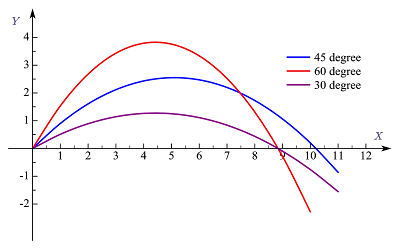# Maximum range of projectile formula

Let us consider a projectile projected with initial velocity $v_{0}$ making an angle $\theta_0$ with the horizontal as shown below in the figure.Projectile Motion

### The maximum range for projectile motion

A projectile of the same mass can be launched with the same initial velocity and different angles $\theta_0$.
Consider the figure given below where a projectile is launched with three different angles $45^0,\,\,60^0,\,\,and\,\,30^0$.A projectile launched at different angles but the same initial velocity
From the above figure, we can clearly see that for different angles of projection horizontal range of projectile is different.
Let us now investigate the angle of projection for which range is maximum (note that initial velocity remains the same).
$R=\frac{v_0 \sin 2\theta_0}{g}$ In above relation, if we keep initial velocity same for various launch , then value of $R$ varies with the value of $\sin 2\theta_0$.
Clearly, from trigonometry, we know that the maximum value of $\sin \theta=1$ and we know that $\sin 90^0=1$
So in this case, we have
$2\theta_0=90^0 \qquad or,\qquad \theta_0=45^0$
Thus the horizontal range of a projectile for a given initial velocity is maximum when it is projected at an angle of $45^0$ with the horizontal.

### The maximum range of projectile formula

To find the formula for a maximum range put $\theta_0=45^0$ in equation (1). So, from equation (1) we have
$R_m=\frac{v_0^2 \sin 90^0}{g}=\frac{v_0^2}{g}$

#### Question That can be asked from this topic

Question 1: Why do 45 degrees give a maximum range of a projectile?
Question 2: Prove 45 degrees maximum range for a projectile launched at an angle $\theta$.
Question 3: Derive the formula for the range of projectile body launched at an angle $\theta$.